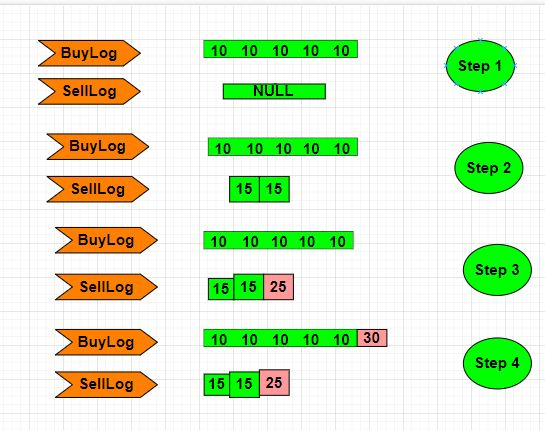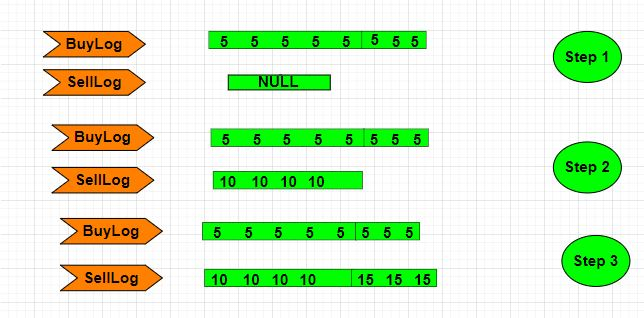1

# NINJA’S KHATA

Difficulty: MEDIUM
Avg. time to solve
15 min
Success Rate
85%

Problem Statement
Suggest Edit

#### Ninja has started working as a trader where he buys a stock and sells them. So ninja has to maintain a ‘KHATA’ where he is keeping entries of the order which are still not executed. Orders are given in the form of the 2-D array where each:

``````‘ORDER_ARR[i] = [RATE[i], AMOUNT[i], TYPE[i]]’  denotes that ‘AMOUNT’ orders have been placed of type ‘TYPE’ at the rate ‘RATE’. The ‘TYPE’ is:
0 represents it as a batch of buy orders, or
1 represents it as a batch of sell orders.
``````

#### The ‘KHATA’ is initially empty. When an order is placed, the following steps take place:

``````If the order is a buy order, you look at the sell order with the smallest price in the ‘KHATA’.
If that sell order's price is smaller than or equal to the current buy order's price, they will match and be executed, and that sell order will be removed from the ‘KHATA’.
If the order is a sell order, you look at the buy order with the largest price in the ‘KHATA’.
If that buy order's price is larger than or equal to the current sell order's price, they will match and be executed, and that buy order will be removed from the ‘KHATA’.
Else, the sell order is added to the ‘KHATA’.
``````

#### Example:``````Suppose the given ‘ORDER_ARR’ is [ [ 10, 5, 0 ], [ 15, 2, 1 ], [ 25, 1, 1 ], [ 30, 4, 0 ] ]
Now 5 orders of type buy with price 10 are placed. There are no sell orders, so the 5 orders are added to the ‘KHATA’.
2 orders of type sell with price 15 are placed. There are no buy orders with prices larger than or equal to 15, so the 2 orders are added to the ‘KHATA’.
1 order of type sell with price 25 is placed. There are no buy orders with prices larger than or equal to 25 in the ‘KHATA’, so this order is added to the ‘KHATA’.
4 orders of type buy with price 30 are placed. The first 2 orders are matched with the 2 sell orders of the least price, which is 15 and these 2 sell orders are removed from the ‘KHATA’. The 3rd order is matched with the sell order of the least price, which is 25 and this sell order is removed from the ‘KHATA’. Then, there are no more sell orders in the ‘KHATA’, so the 4th order is added to the ‘KHATA’.
Finally, the ‘KHATA’ has 5 buy orders with a price of 10, and 1 buy order with a price of 30. So the total number of orders in the ‘khata’ is 6.
``````
##### Note:
``````1. The answer can be large, so return (ans % 10^9+7)
``````

#### Input Format:

``````The first line of input contains a ‘T’ number of test cases.

The first line of each test case contains an integer ‘N’ denoting the number of rows in array ‘ORDER_ARR’. Then, ‘N’ lines follow.

Each line contains three integers denoting the number of columns in that row and ‘3’ space-separated integers denoting the ‘RATE’, ‘AMOUNT’, and ‘TYPE’ value respectively.
``````

#### Output Format:

``````For each test case, print the total amounts of order in the ‘KHATA’.
``````
##### Note:
``````You do not need to print anything, it has already been taken care of. Just implement the given function.
``````

#### Constraints:

``````1 <= ‘T’ <= 10
1 <= ‘N’ <= 1000
1 <= ‘RATE’, ‘AMOUNT’ <=10 ^ 6
‘TYPE’  = [ ‘0’, ‘1’ ]

Where ‘T’ represents the number of test cases and ‘N’ represents the size of ‘ORDER_ARR’.

Time Limit: 1 sec.
``````

#### Sample Input 1:

``````2
4
10 5 0
15 2 1
25 1 1
30 4 0
3
5 8 0
10 4 1
15 3 1
``````

#### Sample Output 1:

``````6
15
``````

#### Explanation For Sample Input 1:

``````Test Case 1:
````````````For the first test case given ‘ORDER_ARR’ is [ [ 10, 5, 0 ], [ 15, 2, 1 ], [ 25, 1, 1 ], [ 30, 4, 0 ] ]
Now 5 orders of type buy with price 10 are placed. There are no sell orders, so the 5 orders are added to the ‘KHATA’.
2 orders of type sell with price 15 are placed. There are no buy orders with prices larger than or equal to 15, so the 2 orders are added to the ‘KHATA’.
1 order of type sell with price 25 is placed. There are no buy orders with prices larger than or equal to 25 in the ‘KHATA’, so this order is added to the ‘KHATA’.
4 orders of type buy with price 30 are placed. The first 2 orders are matched with the 2 sell orders of the least price, which is 15 and these 2 sell orders are removed from the ‘KHATA’. The 3rd order is matched with the sell order of the least price, which is 25, and this sell order is removed from the ‘KHATA’. Then, there are no more sell orders in the ‘KHATA’, so the 4th order is added to the ‘KHATA’.
Finally, the ‘KHATA’ has 5 buy orders with a price of 10, and 1 buy order with a price of 30. So the total number of orders in the ‘KHATA’ is 6.

Test Case 2:
````````````For this test case given ‘ORDER_ARR’ is [ [ 5, 8, 0 ], [ 10, 4, 1 ], [ 15, 3, 1 ] ]
Now 8 orders of type buy with price 5 are placed. There are no sell orders, so the 8 orders are added to the ‘KHATA’.
4 orders of type sell with price 10 are placed. There are no buy orders with prices larger than or equal to 10, so the 4 orders are added to the ‘KHATA’.
3 order of type sell with price 15 is placed. There are no buy orders with prices larger than or equal to 15 in the ‘KHATA’, so this order is added to the ‘KHATA’. So we return ‘15’ as ‘15’ orders are in our ‘KHATA’.
``````

#### Sample Input 2:

``````2
4
23 5 0
35 2 1
45 1 1
50 4 0
3
33 3 0
42 1 1
61 1 1
``````

#### Sample Output 2:

``````6
5
``````
##### Explanation For Sample Input 2:
``````For first test case, total amounts of order in the khata is 6.

For second test case, total amounts of order in the khata is 5.
``````Console